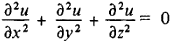# Laplace's Differential Equation

Also found in: Dictionary.

## Laplace’s Differential Equation

the partial differential equationwhere x, y, and z are independent variables and u = u(x, y, z) is an unknown function. The equation was named after P. Laplace, who examined it in works on the theory of gravity (1782). A number of problems in physics and technology reduce to Laplace’s differential equation. It is satisfied by the temperature in stationary processes, the electrostatic field potential at points in space that have no charge, the gravitational field potential in a region lacking gravitational masses, and other quantities. Functions that satisfy Laplace’s differential equation are called harmonic functions.

Site: Follow: Share:
Open / Close